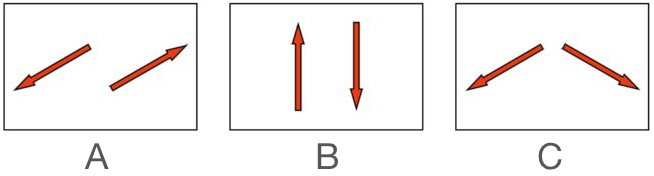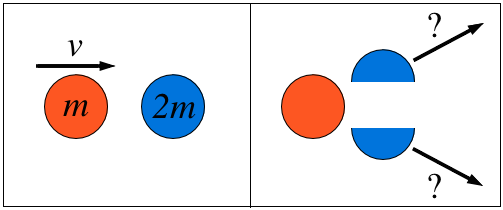Classical Mechanics

# Momentum: Level 1-2 ChallengesTwo billiard balls of equal mass are rolled in such a way that their velocities have the same magnitude but are in opposite directions.

Which of the following is an impossible outcome for the velocities of the billiard balls after a collision?

Note: Collision may or may not be elastic.

You went to a park in winter to practice ice skating on a frozen lake, but because you're a beginner, you asked your friend to give you some impulse to start.

He applied a force of 150 Newtons to you during 1.2 seconds, after which you began moving with a constant velocity.

Another person is skating toward you with a velocity of 5 m/s. Unable to stop, you both collide and both stop moving.

Suppose that you have a mass of 60kg. Find the mass (in kilograms) of the person who collided with you.

Details and assumptions: Because the lake is frozen, the icy surface causes negligible friction.

A cricket ball of mass 150g moving with a speed of 126km/h hits at the middle of the bat, held firmly at its position by the batsman. The ball moves straight back to the bowler after hitting the bat. Assuming that collision between ball and bat is completely elastic and the two remain in contact for 0.001s, what is the magnitude of the average force that the batsman had to apply in order to hold the bat firmly in its place?A body of mass $m$ is moving along the $x$-axis with speed $v$ when it collides with a body of mass $2m$ initially at rest. After the collision, the first body comes to rest and the second body splits into two pieces of equal mass that move with equal velocities at equal angles off the $x$-axis $0 < \theta < 90^\circ$, as shown in the figure.

Which of the following statements correctly describes the speeds of the two pieces?

Mr. Andrew excites his students with his home-made cannon demonstration. The 1.27kg cannon is loaded with a 54 grams cricket ball and placed on the ground. Mr. Andrew fires the cannonball horizontally and determines that the cannon recoiled backwards with a speed of 7.8m/s with help of a photogate measurement and asks his students to determine the speed of the ball. So, what is the speed of the ball in m/s?

×